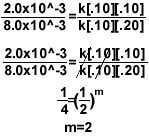MainTutorialsOrganic ChemistryPractice TestsOnline QuizzesReference ToolsKinetics Practice Questions
Answer each question on your own paper. The answers have been provided below. Remember, you can only learn from your mistakes! Better to make those mistakes here than on your tests.

Use these two graphs to answer question 1.1.) Find the reaction rate of the decomposition of methane between 3 and 7 seconds.

1b.) Find the reaction rate of the production of oxygen gas between 2 and 6 seconds.

1c.) Identify the reactant and product based on the graph.

2.) How does temperature affect reaction rate?

3.) What is activation energy?

4.) Explain how a catalyst works relative to activation energy.

5.) With the given information, determine the rate law, the rate constant, and the overall reaction order.

 2Mg + O2 Ţ 2MgO Rate = k[Mg]n[O2]m Trial Initial [Mg] (mol/L) Initial [O2] (mol/L) Measured Rate (mol/L*s) 1 .10 .10 2.0x10^-3 2 .20 .10 4.0x10^-3 3 .10 .20 8.0x10^-3

6a.) Find the half-life of a first-order reaction if the reaction constant, k , is 2.0x10^-3 s-1.

6b.) Find the time when only 1% of reactant remains.

7.) Find  Ea using the following information:

ClO3- + H2O Ţ ClO4- + H2

 Reaction constant k, (s-1) Temperature(C° ) 2.0x10^-3 25 4.0x10^-3 35 8.0x10^-3 45 1.6x10^-2 558.) Find the 2nd order reaction’s activation energy with the given information.

 K1=4.0L/mol*s at 37C° K2=8.0L/mol*s at 87C°

Hint:1.)

• The reaction rate is simply: the change in amount of something / D T.
• I know the rate law is: D [A] / D T, where [A] is the molarity.

17-35= -18

-18/4= -4.5 L/t

1b.)

• The reaction rate is simply: the change in amount of something / D T.
• I know the rate law is: D [A] / D T, where [A] is the molarity.

36ml-13ml / 6-3

23ml/3s = 7.7 ml/s

1c.)

• Oxygen would be the product because its reaction rate graph has a positive slope; the concentration of oxygen is increasing.
• Methane would be the reactant because its reaction rate graph has a negative slope; the concentration of methane is decreasing.

2.) Temperature increased the reaction rate. Remember for a reaction to take place, the molecules must move faster. An increase in temperature speeds up the molecules.

3.) Activation energy is the minimum amount of energy the reaction must overcome in order for the reaction to take place.

4.) A catalyst lowers the activation energy; therefore, increases the reaction rate.

5.)• To find the order for Mg, setup the equation like above so the concentration of oxygen cancels out.
• To find the order for O2, simply try to cancel the concentration of Mg.
• For the rate constant, once you have the rate law, plug in any row of data from the chart and solve for k. Make sure the concentration corresponds to the measured rate.

2.0x10^-3=k[.10]1[.10]2

k=2.0

Final rate law = k[Mg]1[O2]2

Over all reaction order = n + m= 3

6a). Because it is a first-order reaction, the half-life equation is ln2 / k.

ln2/ 2.0x10^-3 = 350 seconds.

I know the unit is seconds because the unit of my reaction constant is s s-1.

*If the unit is minute-1, the half life would be 350 minutes.

6b) The integrated rate law of a first order-reaction is: ln[A]=-kt+ln[A]07.)

Another way of looking at this equation is: y=mx+b. Looks familiar? It’s the equation of a line.

y=lnk; m=-Ea/R; x=1/T; b= ln(A).

slope =-Ea/R

Find the slope of the line when graphing lnk vs. 1/T.

(.00325-.00336)/(-5.53+ 6.21)= -6270

Remember R= 8.3145 J and convert T to kelvin.

-6270=-Ea/R

-6270*-8.3145= 5.21x10^4 J/mol

8.)

Make sure you change the temperature to kelvin.

37C° =300k

87C° =360kR= 8.3145 Jnews | about us | contact us tutorials index | organic chemistry | practice tests | online quizzes | reference tools site copyright (c) 2002-2013 Learn ChemSubscribe to our low volume newsletter to receive up-to-date information about the CHEM SITE